GATE Chemistry Mock Test - 1

# GATE Chemistry Mock Test - 1 - Chemistry

Test Description

## 65 Questions MCQ Test GATE Chemistry Mock Test Series - GATE Chemistry Mock Test - 1

GATE Chemistry Mock Test - 1 for Chemistry 2023 is part of GATE Chemistry Mock Test Series preparation. The GATE Chemistry Mock Test - 1 questions and answers have been prepared according to the Chemistry exam syllabus.The GATE Chemistry Mock Test - 1 MCQs are made for Chemistry 2023 Exam. Find important definitions, questions, notes, meanings, examples, exercises, MCQs and online tests for GATE Chemistry Mock Test - 1 below.
Solutions of GATE Chemistry Mock Test - 1 questions in English are available as part of our GATE Chemistry Mock Test Series for Chemistry & GATE Chemistry Mock Test - 1 solutions in Hindi for GATE Chemistry Mock Test Series course. Download more important topics, notes, lectures and mock test series for Chemistry Exam by signing up for free. Attempt GATE Chemistry Mock Test - 1 | 65 questions in 180 minutes | Mock test for Chemistry preparation | Free important questions MCQ to study GATE Chemistry Mock Test Series for Chemistry Exam | Download free PDF with solutions
 1 Crore+ students have signed up on EduRev. Have you?
GATE Chemistry Mock Test - 1 - Question 1

### She has a sharp tongue and it can occasionally turn____________:

GATE Chemistry Mock Test - 1 - Question 2

GATE Chemistry Mock Test - 1 - Question 3

### In the summer, water consumption is known to decrease overall by 25%. A water board official states that in the summer household consumption decreases by 20% while other consumption increases by 70% Which of the following statements is correct?

GATE Chemistry Mock Test - 1 - Question 4

40% of deaths on city roads may be attributed to drunken driving. The number of degrees needed to represent this as a slice of a pie chart is:

GATE Chemistry Mock Test - 1 - Question 5

Some tables are shelves. Some shelves are chairs. All chairs are benches. Which of the following conclusions can be deduced from the preceding sentences?
(I) At least one bench is a table  (II) At least one shelf is a bench
(III) At least one chair is a table  (IV) All benches are chairs

GATE Chemistry Mock Test - 1 - Question 6

“If you are looking for a history of India, or an account of the rise and fall of the British Raj, or for the reason of the cleaving of the subcontinent into two mutually antagonistic parts and the effects this mutilation will have in the respective sections, and ultimately on Asia, you will not find it in these pages; for though I have spent a lifetime in the country. I lived too near the seat of events, and was too intimately associated with the actors, to get the perspective needed for the impartial recording of these matters.” Here, the word ‘antagonistic’ is closest in meaning to:

GATE Chemistry Mock Test - 1 - Question 7

Trucks (10 m long) and cars (5 m long) go on a single lane bridge. There must be a gap of at least 20 m after each truck and a gap of at least 15 m after each car. Trucks and cars travel at a speed of 36 km/h. If cars and trucks go alternately. What is the maximum number of vehicles that can use the bridge in one hour?

GATE Chemistry Mock Test - 1 - Question 8

There are 3 Indians and 3 Chinese in a group of 6 people. How many subgroups of this group can we choose so that every subgroup has at least one Indian?

GATE Chemistry Mock Test - 1 - Question 9

The velocity V of a vehicle along a straight line is measured in m/s and plotted as shown with respect to time in seconds. At the end of the 7 seconds, how much will the odometer reading increase by (in m)?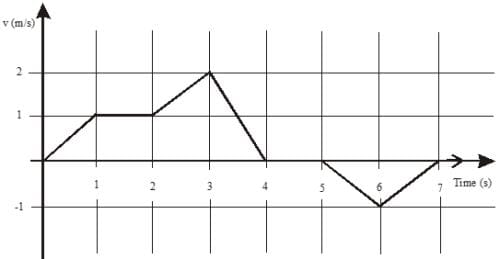GATE Chemistry Mock Test - 1 - Question 10

A cube of side 3 units is formed using a set of smaller cubes of side 1 unit. Find the proportion of the number of faces of the smaller cubes visible to those which are not visible:

GATE Chemistry Mock Test - 1 - Question 11

Which of the following metallocene has 3.87 BM value of magnetic moment?

GATE Chemistry Mock Test - 1 - Question 12

Consider the compounds PF5, SbF5, PH3 and SbH3. The strongest acid and strongest base among these are resp:

GATE Chemistry Mock Test - 1 - Question 13

Which of the following is true for 2px, 2py and 2pz orbitals of a H-atom?

GATE Chemistry Mock Test - 1 - Question 14

The species which by definition has zero standard molar enthalpy of formation at 298 K is:

GATE Chemistry Mock Test - 1 - Question 15

In fcc crystal lattice, edge length is 400 pm. Find the diameter of greatest sphere which can be fitted into the interstitial void without distortion of lattice:

GATE Chemistry Mock Test - 1 - Question 16

The molecules with the smallest rotation partition function at any temperature among the following is:

GATE Chemistry Mock Test - 1 - Question 17

Which statement is/are correct?

GATE Chemistry Mock Test - 1 - Question 18

Choose incorrect statement:

GATE Chemistry Mock Test - 1 - Question 19

At room temperature, which molecule has the minimum rotational entropy?

GATE Chemistry Mock Test - 1 - Question 20

Predict the product for the following sugar functionalization reaction: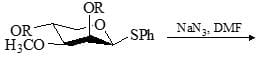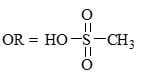GATE Chemistry Mock Test - 1 - Question 21

Consider the following: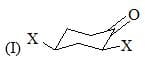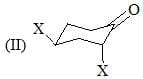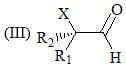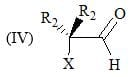Order of their stretching frequency in IR-spectroscopy would be:

GATE Chemistry Mock Test - 1 - Question 22

1H NMR spectrum of an organic compound recorded on a 500 MHz spectrometer showed a quartet with line positions at 1759, 1753, 1747, 1741 Hz. Chemical shift (s) and coupling constant (Hz) of the quartet are?

GATE Chemistry Mock Test - 1 - Question 23

Choose incorrect option in reference to symmetry elements present in ‘Ethane (staggered)’ molecule:

GATE Chemistry Mock Test - 1 - Question 24

The standard emf of the cell, Cd(s) | CdCl2(aq) (0.1 M) || AgCl(s) | Ag(s) in which the cell reaction, is,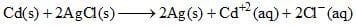is 0.6915 V at 0°C and 0.6753 V at 25°C. The DH° of the reaction at 25°C is:

GATE Chemistry Mock Test - 1 - Question 25

Choose the correct product of the following reaction: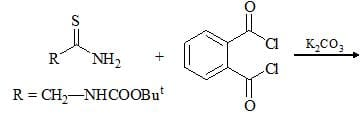GATE Chemistry Mock Test - 1 - Question 26

The increasing order of pKa values of the circled hydrogen in the following compounds is?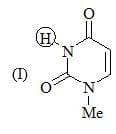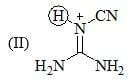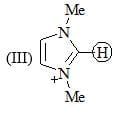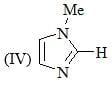GATE Chemistry Mock Test - 1 - Question 27

Consider the compound [M(h6-C6H6)(CS)2]2 as a stable compound which follows 18 e rule. Hence, metal ‘M’ can be

GATE Chemistry Mock Test - 1 - Question 28

The nodal plane in the π-bond of ethene is located in:

GATE Chemistry Mock Test - 1 - Question 29

An inventor claims to have constructed an engine that has an efficiency of 75% when operated between the boiling and freezing points of water. Which of the following is true?

GATE Chemistry Mock Test - 1 - Question 30

Which of the following statement(s) is/are true about the reaction given below?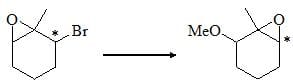GATE Chemistry Mock Test - 1 - Question 31

For the conversion of X to Y, the appropriate sequence of reaction is: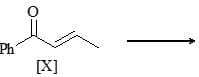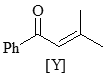GATE Chemistry Mock Test - 1 - Question 32

The g values for Ce3+ (4f1 ) and Pr3+ (4f2 ) are, respectively:

*Answer can only contain numeric values
GATE Chemistry Mock Test - 1 - Question 33

An electronic state of a hydrogen atom is prepared to be a superposition energy of the eigenstates of the hydrogen atom, and for which the normalized wavefunction is written as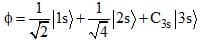Here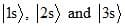are the exact eigen states of the hydrogen atom, and C3s is a constant. What would be the average value of energy, associated with this wave function f, in a.u.:

*Answer can only contain numeric values
GATE Chemistry Mock Test - 1 - Question 34

The sum of s & p bonds in C6N6O3 (Benzotrifurazan):

*Answer can only contain numeric values
GATE Chemistry Mock Test - 1 - Question 35

A plant virus is found to consist of uniform cylindrical particles of 150 angstrom in diameter and 5000 angstrom long. The specific volume of the virus is 0.75 cm3/g. If the virus is considered to be a single particle, find its molar mass (in g/mol, rounded up to three decimal places, in the multiple of 107):

GATE Chemistry Mock Test - 1 - Question 36

Product [B] formed in the following reaction would be: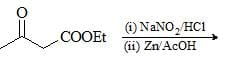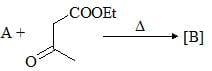GATE Chemistry Mock Test - 1 - Question 37

The point group of Mn2(Co)10 is:

GATE Chemistry Mock Test - 1 - Question 38

Thermal stability of hydrides of first group elements follows the order

GATE Chemistry Mock Test - 1 - Question 39

The values of l and m for the spherical harmonics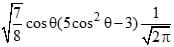would be resp:

GATE Chemistry Mock Test - 1 - Question 40

Choose correct:

GATE Chemistry Mock Test - 1 - Question 41

For the detection of sulfur in an organic compound, Lassaigne’s extract is acidified with acetic acid. Lead acetate solution is then added to it. The black precipitate formed is due to the formation of ?

GATE Chemistry Mock Test - 1 - Question 42

In Monsanto acetic process shown below, the role of HI is: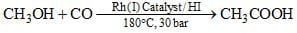GATE Chemistry Mock Test - 1 - Question 43

Each of the compounds Pt(NH3)6Cl4, Cr(NH3)6Cl3, Co(NH3)4Cl3 and K2PtCl6 were dissolved in water to make its 0.001 M solution. The correct order of their increasing conductivity in solution is:

GATE Chemistry Mock Test - 1 - Question 44

Correct reaction among the following is/are: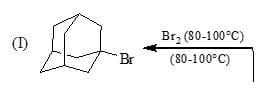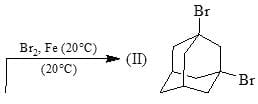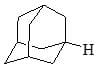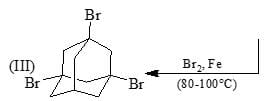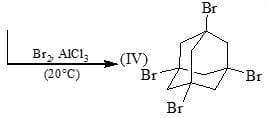GATE Chemistry Mock Test - 1 - Question 45

The spin-only (μS) and spin plus orbital (μS+L) magnetic moment of [CrCl6]3– are:

GATE Chemistry Mock Test - 1 - Question 46

Choose the correct product of the following reaction: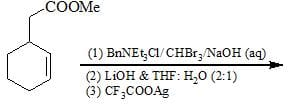GATE Chemistry Mock Test - 1 - Question 47

Choose the correct product of the following reaction: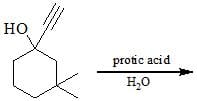GATE Chemistry Mock Test - 1 - Question 48

For opposing reaction, A + B ↔ C + D, Ea = 100 kJ/mol and A = 1010 M–1s–1. The equilibrium concentration of A, B, C, D are 1, 2, 5, 4 M resp., at 700K. The values of k1 and k–1 at this temp are:

GATE Chemistry Mock Test - 1 - Question 49

A, B, C and D elements form compounds AC, A2D and BD. If AC and A2D are radioactive and BD is not radioactive compound, find the following radioactive compounds:
(I) A2   (II) A2C  (III) C2D   (IV) BC

GATE Chemistry Mock Test - 1 - Question 50

The true statement for a polarographic measurement procedure is:

GATE Chemistry Mock Test - 1 - Question 51

The correct order of boiling point is?

GATE Chemistry Mock Test - 1 - Question 52

0.5 mole of each of H2, SO2 and CH4 are kept in a container. A hole was made in the container. After 3 hours, the order of partial pressures in the container will be:

GATE Chemistry Mock Test - 1 - Question 53

Consider the following chemical reaction & predict the major product. Major product has two geometrical isomers which are inter-convertible in presence of light: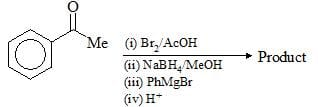*Answer can only contain numeric values
GATE Chemistry Mock Test - 1 - Question 54

Each Rubidium halide crystallizing in the NaCl type lattice has a unit cell length 30 pm greater than for corresponding potassium salt (RK+ = 133 pm) of the same halogen. What is ionic radius (pm) of Rb+________? [Rounded up to two decimal places]

*Answer can only contain numeric values
GATE Chemistry Mock Test - 1 - Question 55

What would be the most probable radius (pm) at which an electron will be found when it occupies a 1s orbital of a Ne9+ atom ________? [Rounded up to two decimal places]

*Answer can only contain numeric values
GATE Chemistry Mock Test - 1 - Question 56

Consider the following reaction: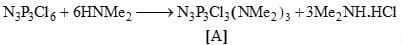The number of possible isomers for [A] is are______?

*Answer can only contain numeric values
GATE Chemistry Mock Test - 1 - Question 57

What would be the relative population of the J = 1 and J = 0 rotational states of HCl at 25°C_______?
(Given: BHCl = 10.591 cm–1) [Rounded up to first decimal place]

*Answer can only contain numeric values
GATE Chemistry Mock Test - 1 - Question 58

For the first order reaction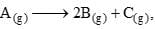the initial pressure is PA = 90 mm Hg, the pressure after 10 minutes is found to be 180 mm Hg. The rate constant (in sec–1)of the reaction is Q × 10–3: Therefore, Q is­­­ __? [Rounded up to two decimal places]

*Answer can only contain numeric values
GATE Chemistry Mock Test - 1 - Question 59

2 mole 'He' is mixed with 2 gm of H2. The molar heat capacity (in the multiple of R) at constant pressure for mixture is_____? [Rounded up to two decimal places]

*Answer can only contain numeric values
GATE Chemistry Mock Test - 1 - Question 60

Ionic radii of Cs+ and Cl ions are 181 pm and 167 pm, respectively. The Born exponents for the He, Ne, Ar, Kr and Xe configurations are 5, 7, 9, 10 and 12 respectively. If the value of ANe2/4ᴨɛo is 2.45×10–4Jm, the lattice energy (in kJ/mol) of CsCl according to Born-Lande equation is______? [Rounded up to first decimal place]

GATE Chemistry Mock Test - 1 - Question 61

On the basis of data given below predict which of the following gases shows least adsorption on a definite amount of charcoal?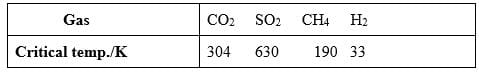GATE Chemistry Mock Test - 1 - Question 62

Choose the correct statement(s) in reference to simple harmonic and anharmonic oscillator model?
(I) Two curves differ widely in lower and higher inter-nuclear distance region
(II) The actual energy diagram rises more steeply than harmonic parabola energy diagram in lower inter-nuclear distance region
(III) In the region near to equilibrium inter-nuclear distance, the curves do not show appreciable departure
(IV) In higher inter-nuclear distance region, rise for actual energy diagram is less steep

GATE Chemistry Mock Test - 1 - Question 63

Consider (I) I, (II) Se2–, (III) Br, (IV) O2–, (V) F and arrange them in decreasing order of ionic radius.

GATE Chemistry Mock Test - 1 - Question 64

Choose the correct product of the following reaction: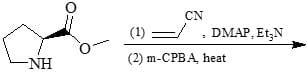GATE Chemistry Mock Test - 1 - Question 65

Consider the following chemical reaction sequence. Predict the product ‘Z’: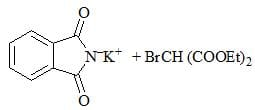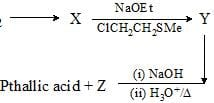## GATE Chemistry Mock Test Series

17 docs|34 tests
Information about GATE Chemistry Mock Test - 1 Page
In this test you can find the Exam questions for GATE Chemistry Mock Test - 1 solved & explained in the simplest way possible. Besides giving Questions and answers for GATE Chemistry Mock Test - 1, EduRev gives you an ample number of Online tests for practice

17 docs|34 tests